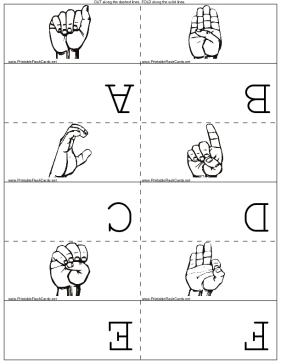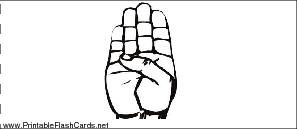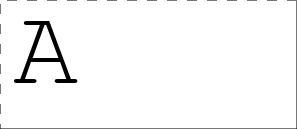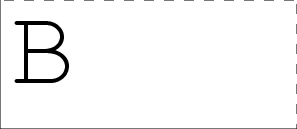Sample flash cards in this set:

Front:Back:This set includes the following cards:
(Image)A
(Image)B
(Image)C
(Image)D
(Image)E
(Image)F
(Image)G
(Image)H
(Image)I
(Image)J
(Image)K
(Image)L
(Image)M
(Image)N
(Image)O
(Image)P
(Image)Q
(Image)R
(Image)S
(Image)T
(Image)U
(Image)V
(Image)W
(Image)X
(Image)Y
(Image)Z